## CrackMe-007## 160CrackMe-07

### 0x01 信息收集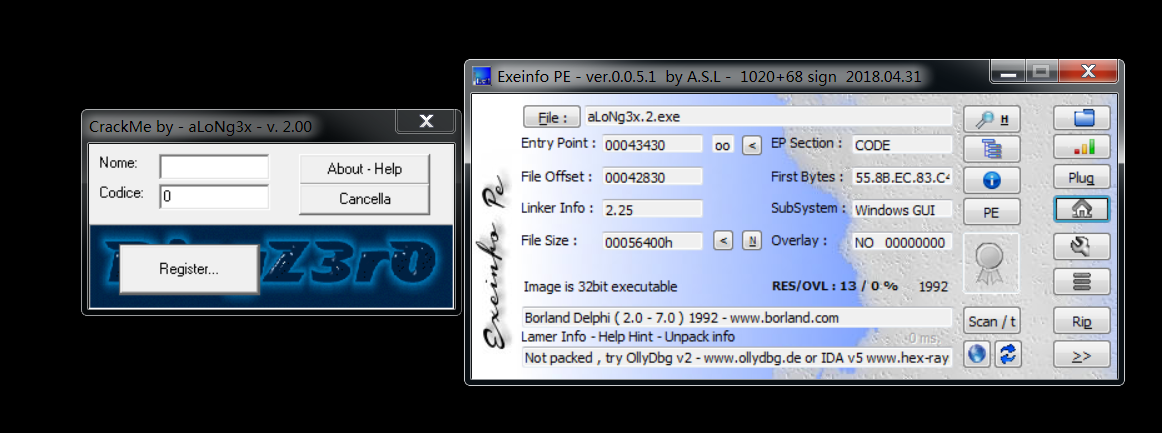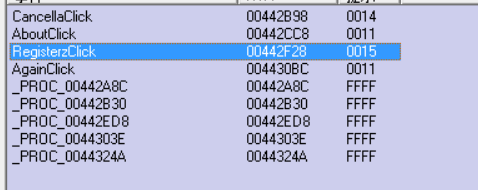• CancellaClick
• RegisterzClick
• AgainClick

### 0x02 爆破与算法破解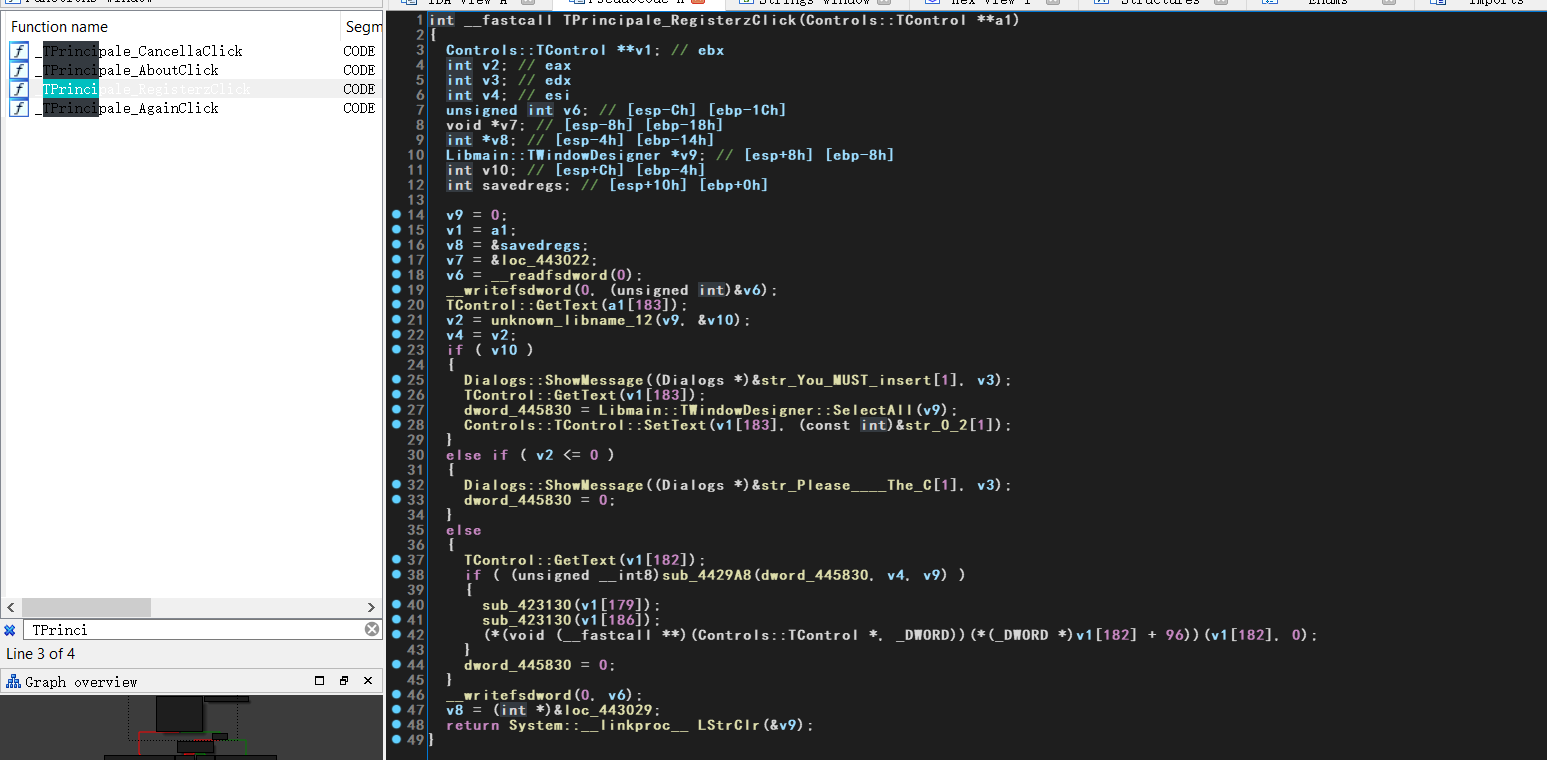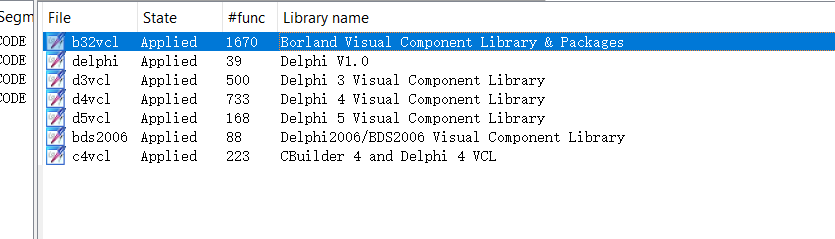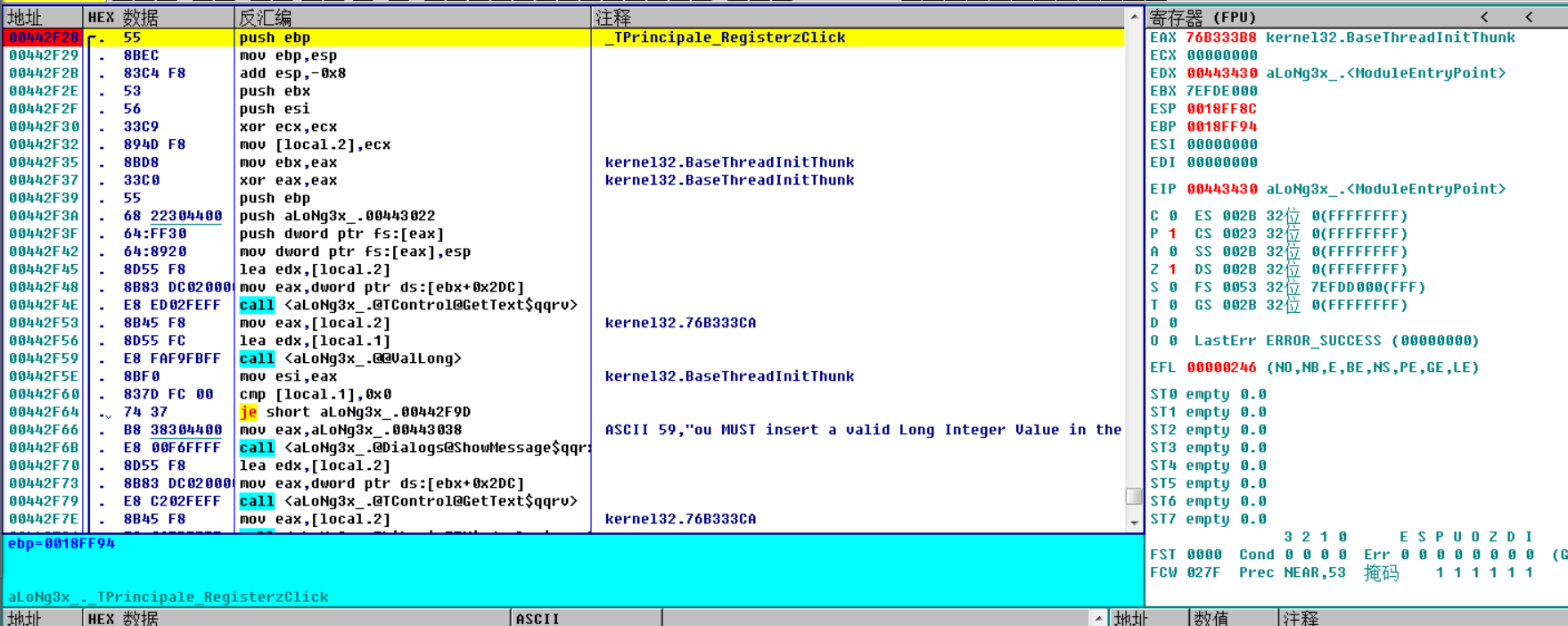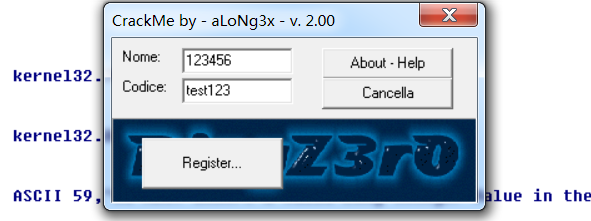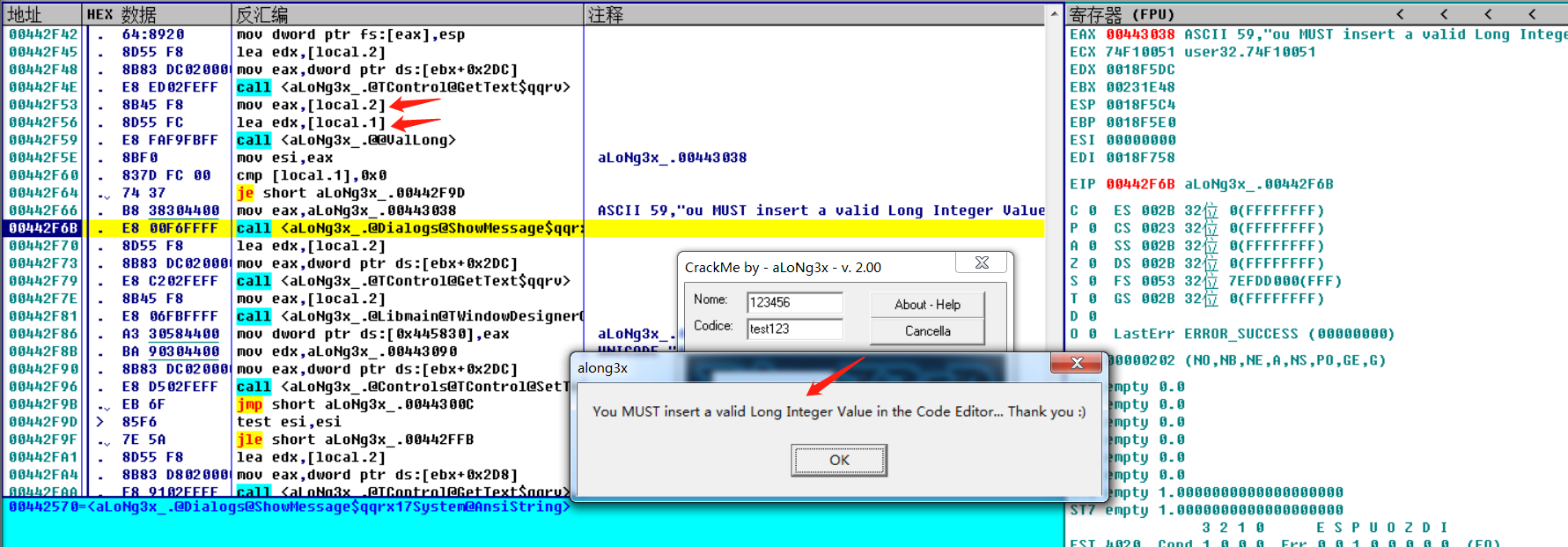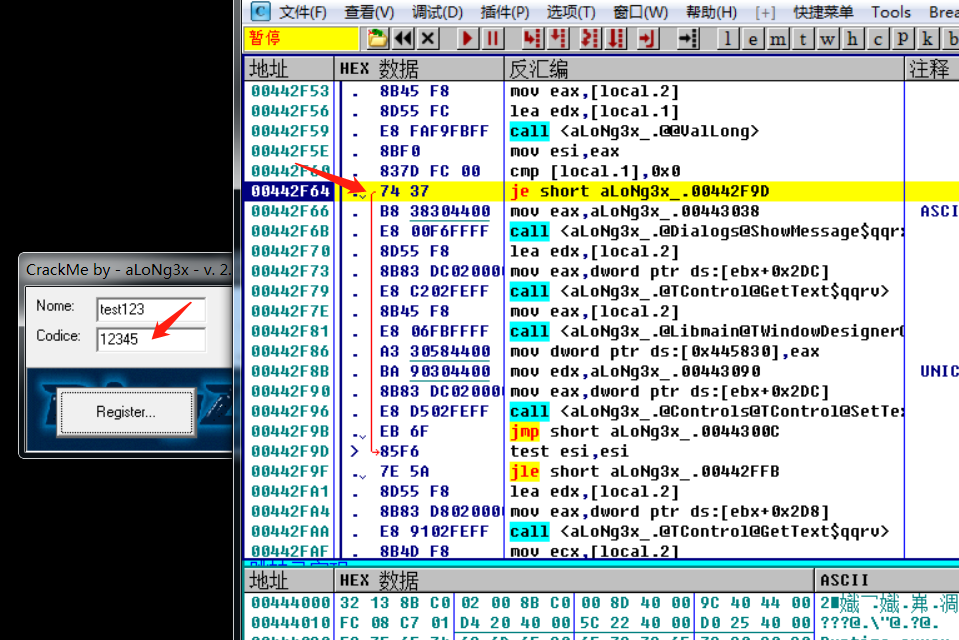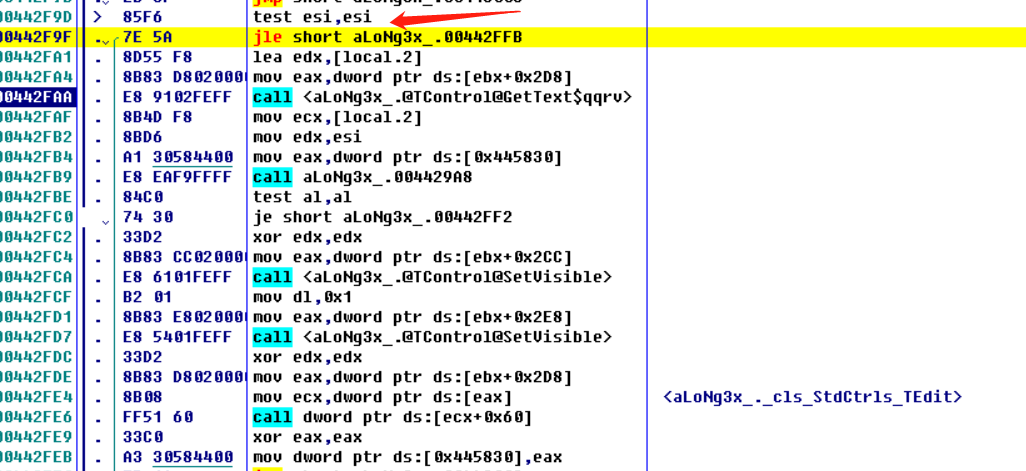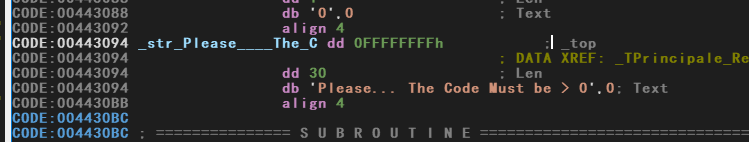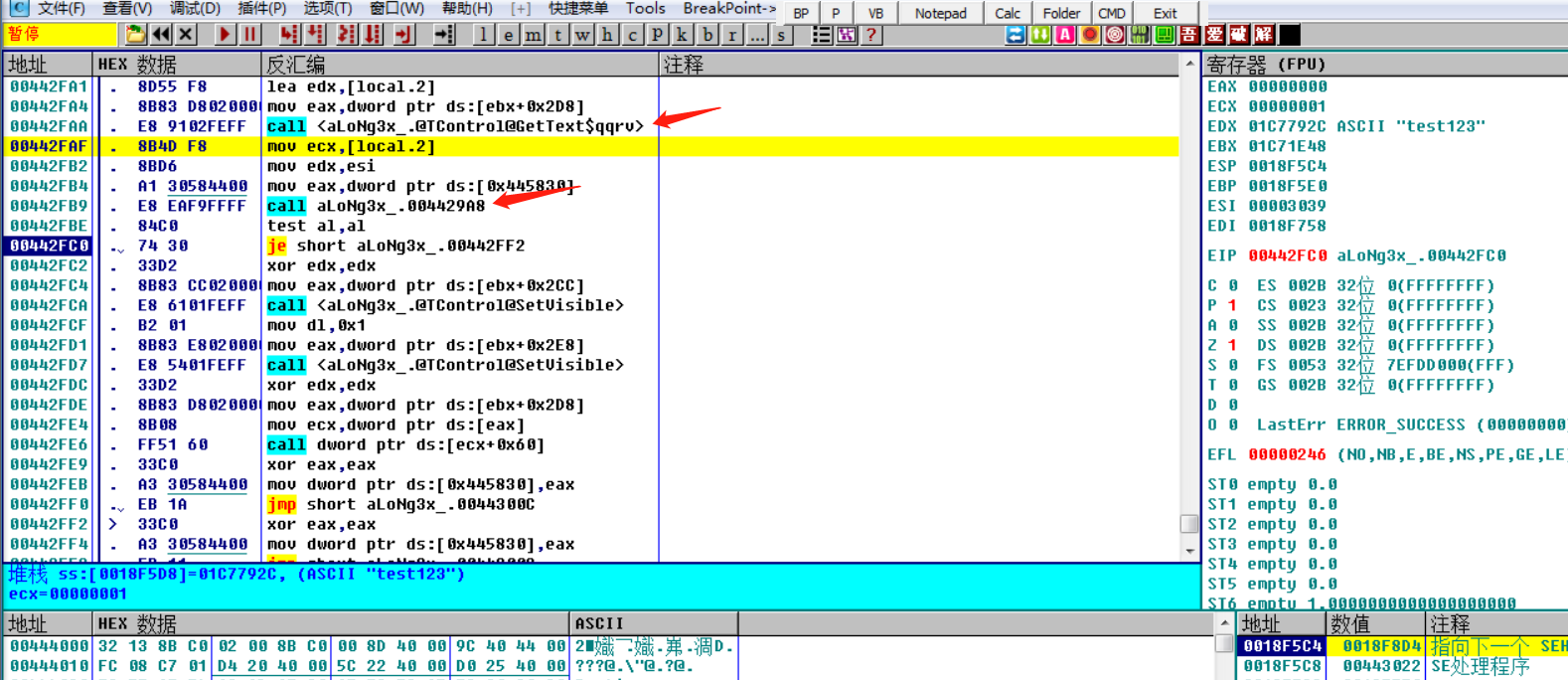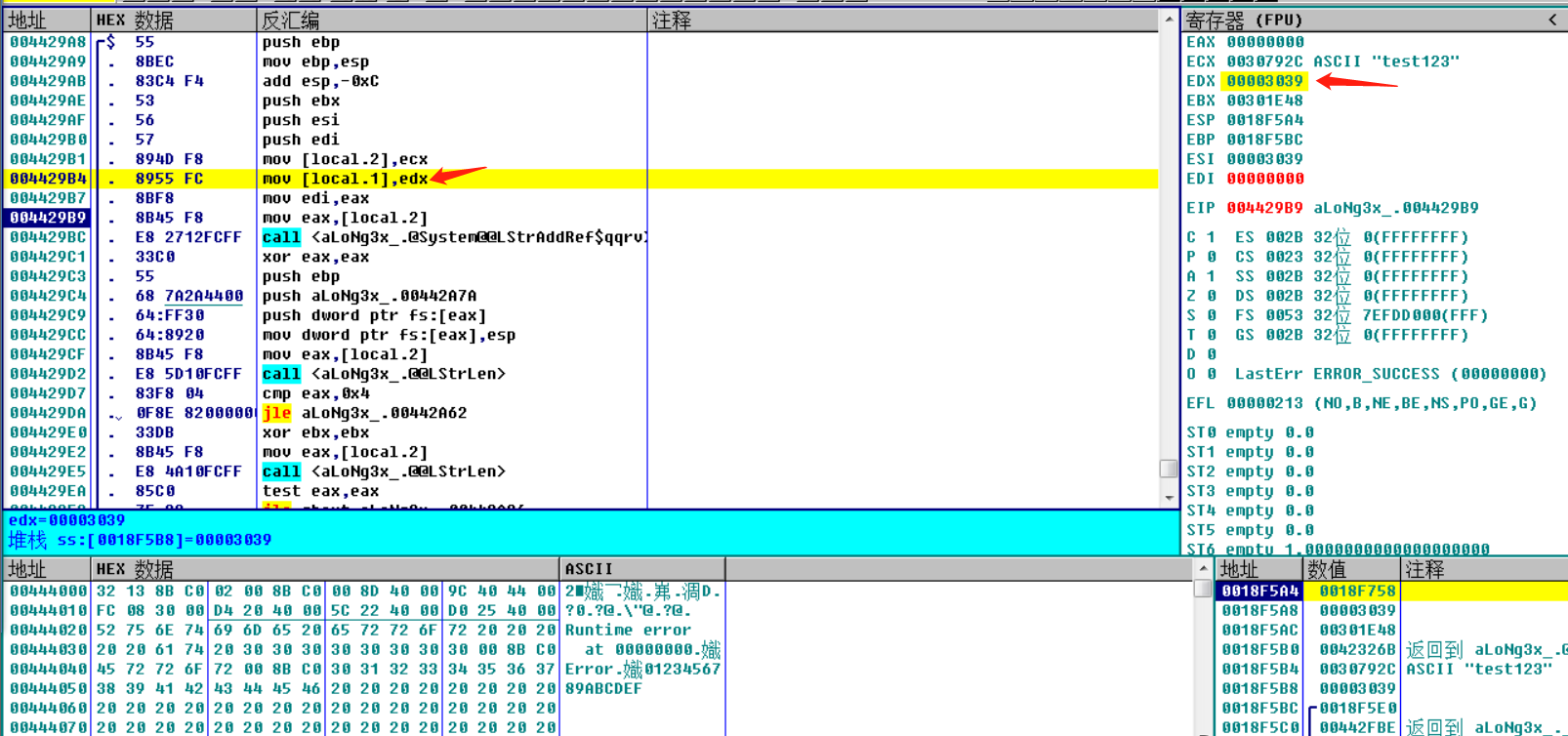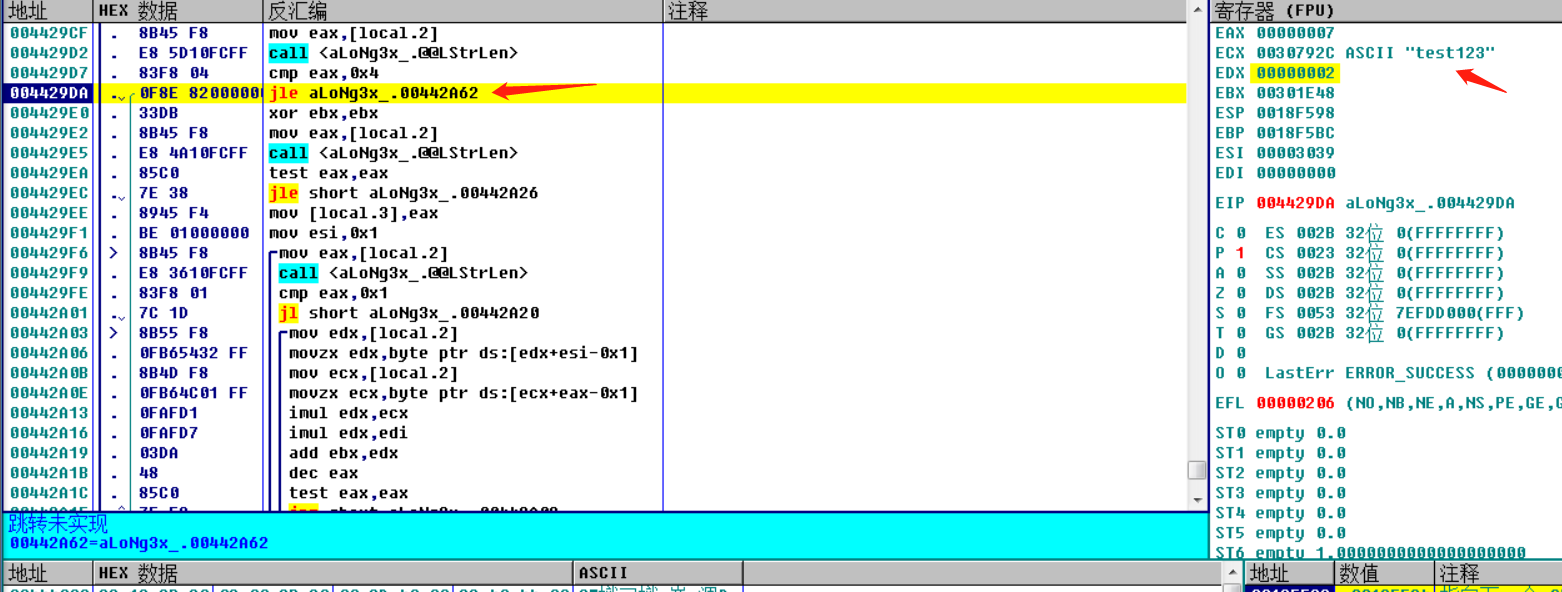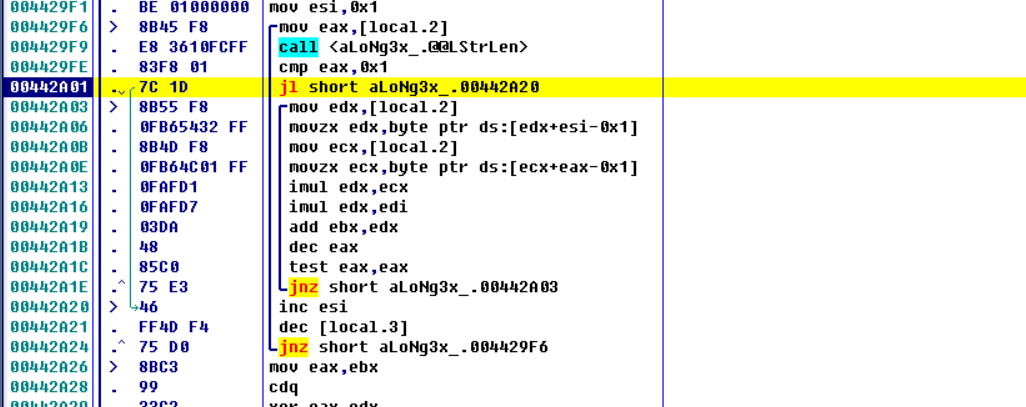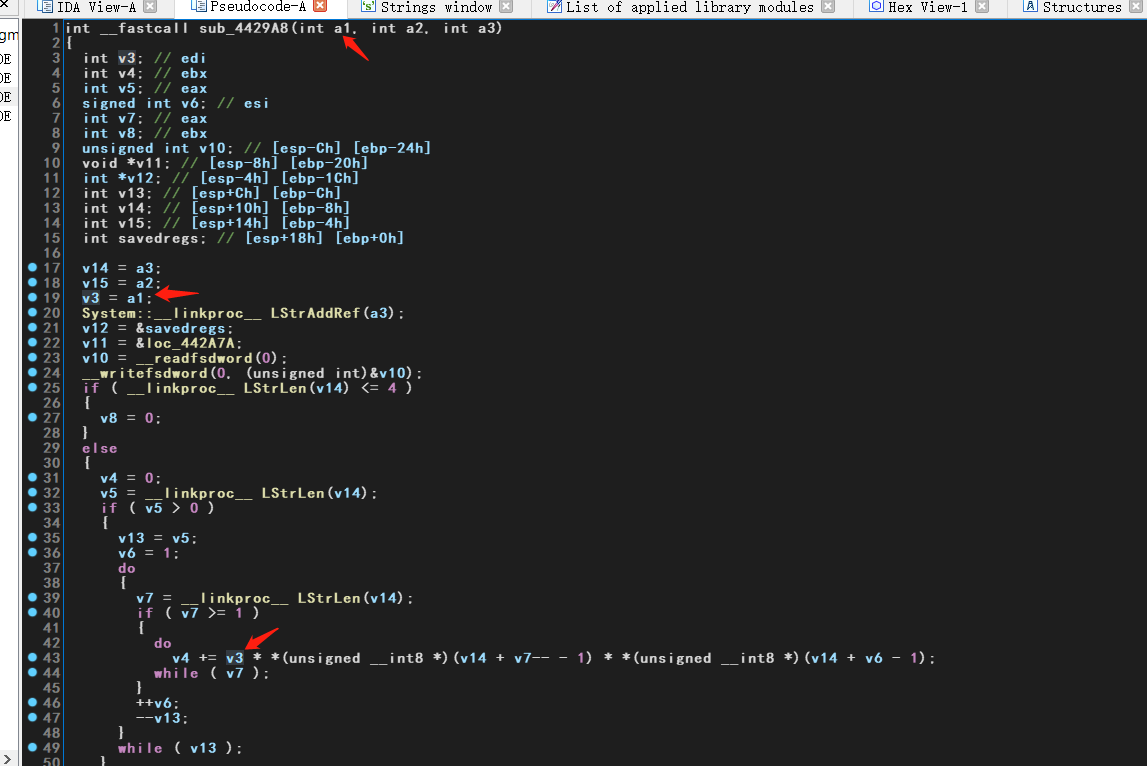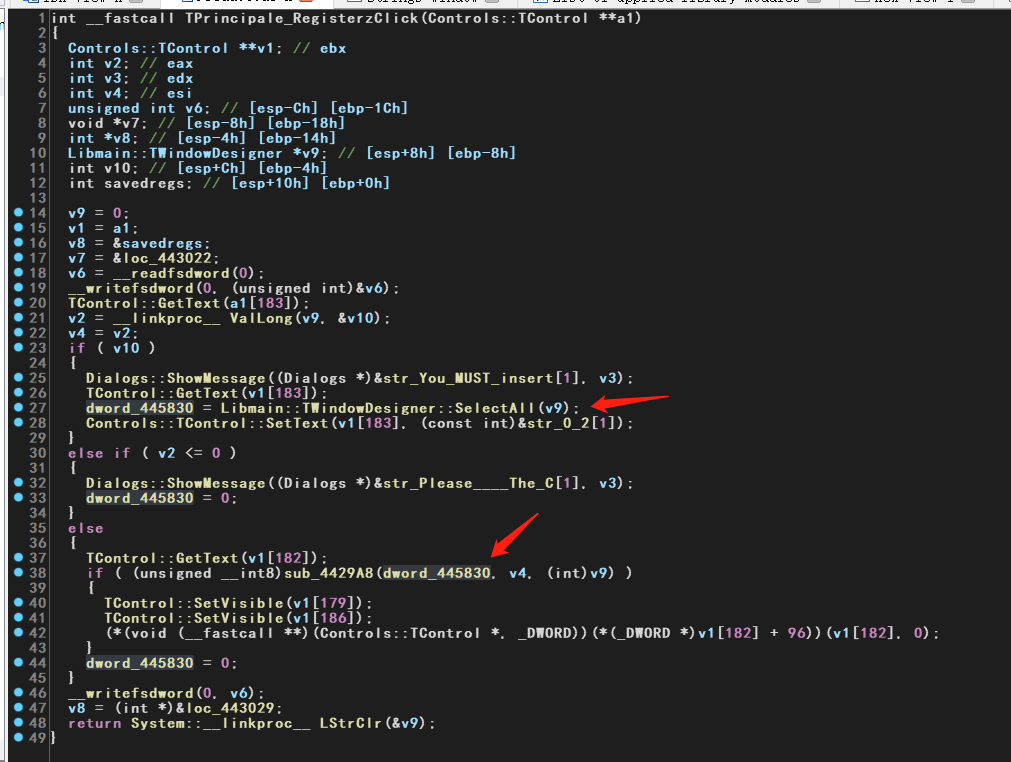nop掉jcc指令，往下看到dword_445830的值还是0：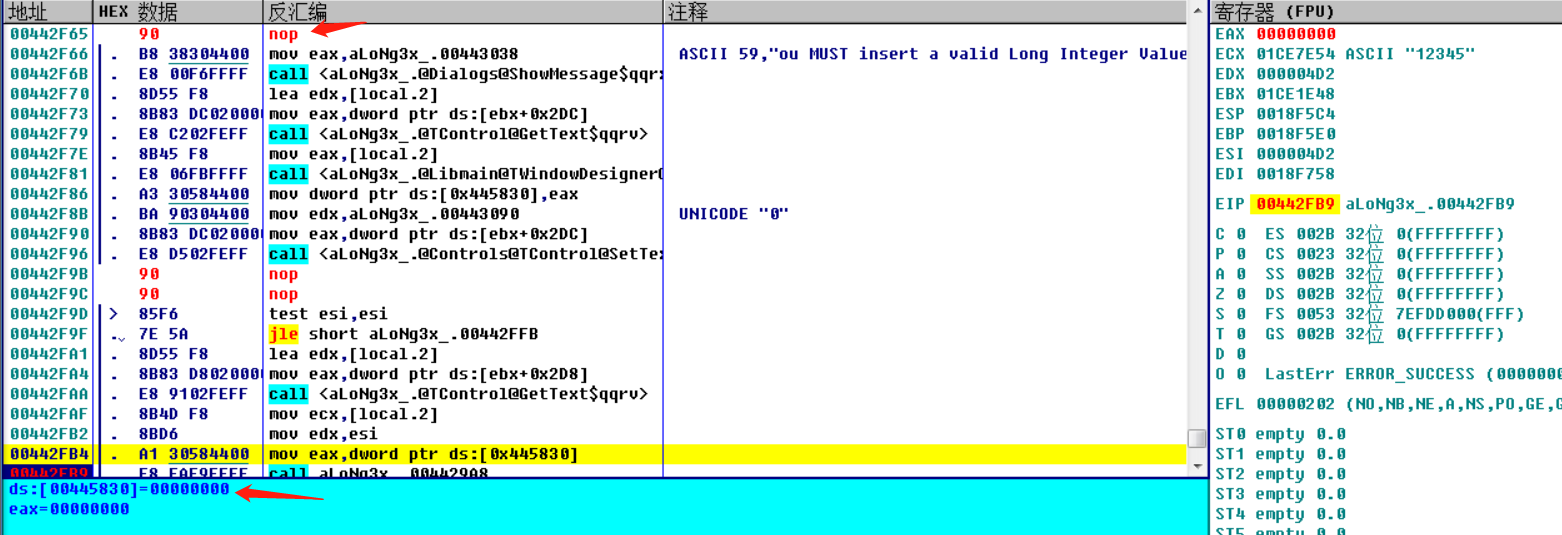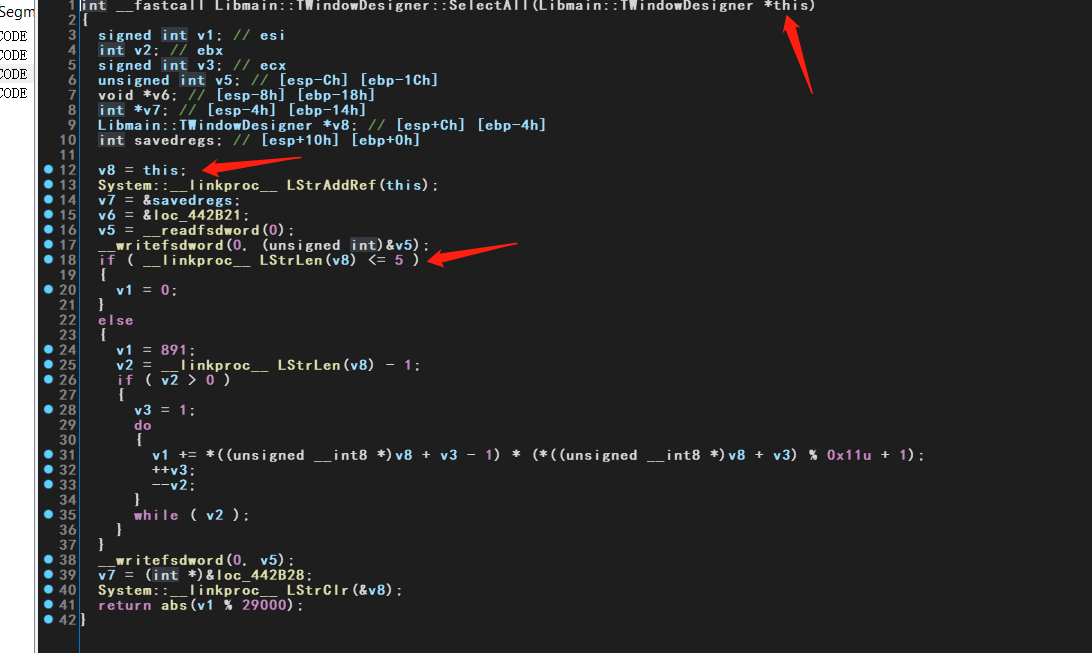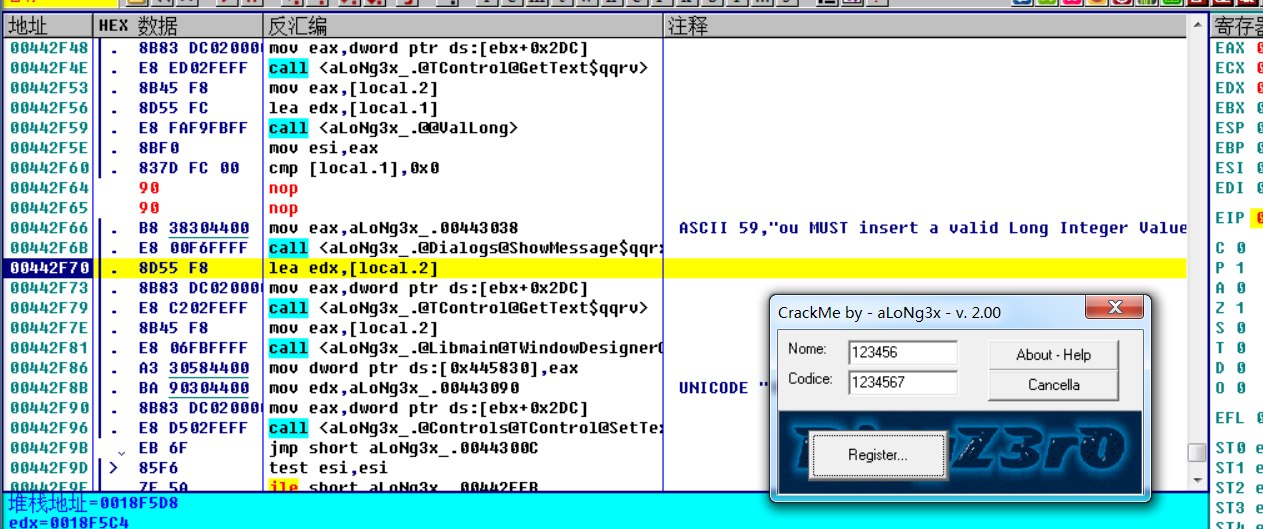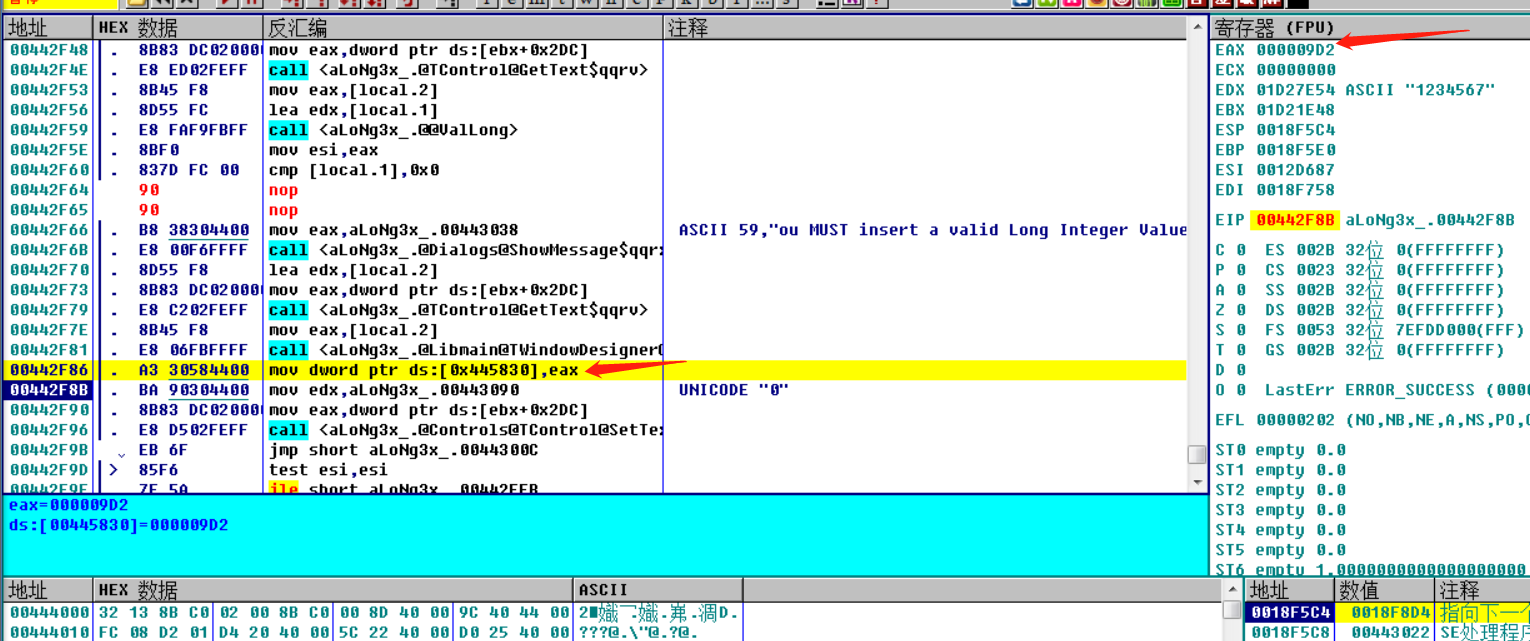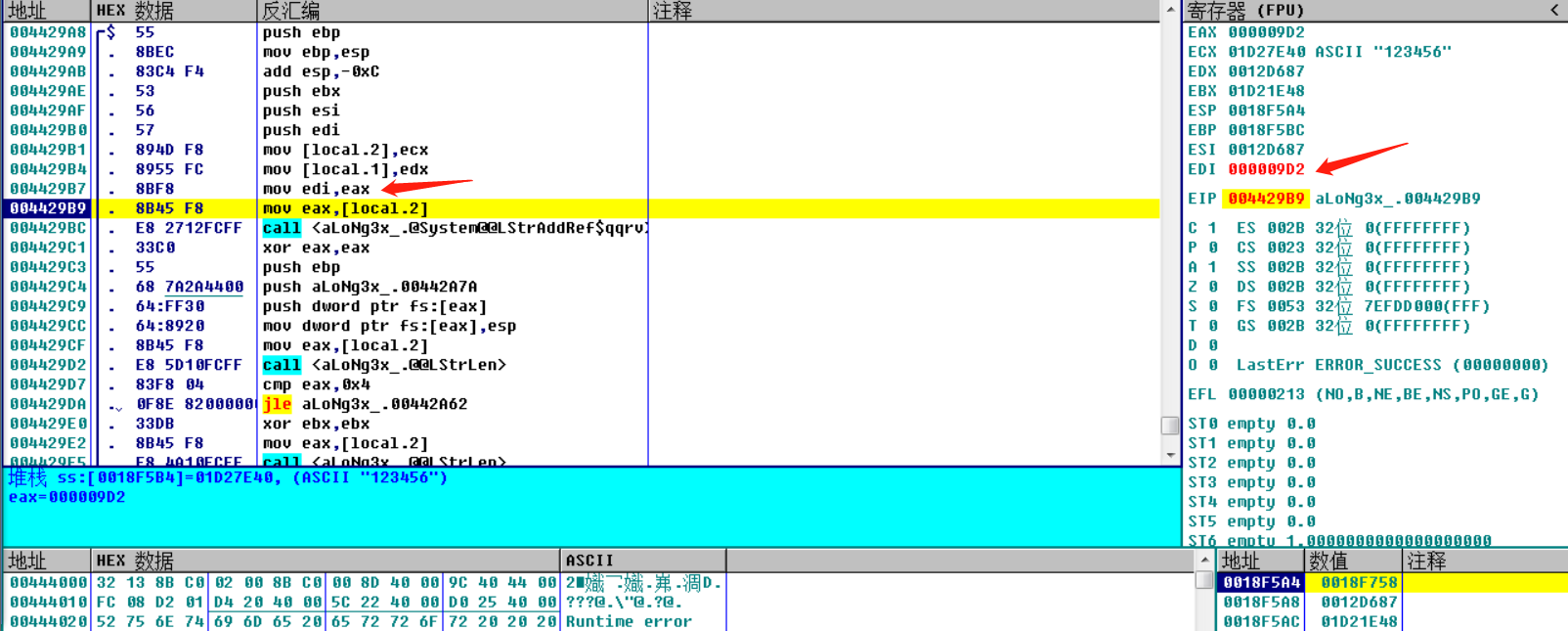``````Codice = '234567'
EDI = 0x37B
for i in range(1, len(Codice)):
EDI += (ord(Codice[i]) % 0x11 + 1) * ord(Codice[i-1])
``````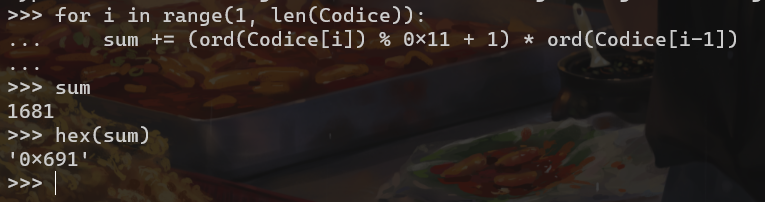``````Nome = '12345'
res = []
for i in Nome:
index = len(Nome) - 1
while index >= 0:
res.append(ord(i) * ord(Nome[index]))
index -= 1
``````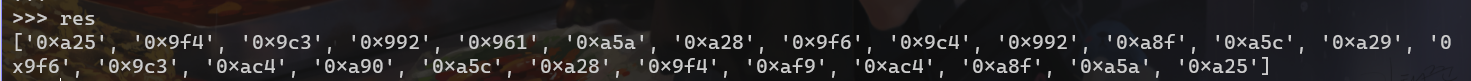0x31 0x32 0x33 0x34 0x35
0x31 0x961 0x992 0x9C3 0x9F4 0xA25
0x32 0x992 0x9C4 0x9F6 0xA28 0xA5A
0x33 0x9C3 0x9F6 0xA29 0xA5C 0xA8F
0x34 0x9F4 0xA28 0xA5C 0xA90 0xAC4
0x35 0xA25 0xA5A 0xA8F 0xAC4 0xAF9

``````Nome = '12345'
sum = 0
EDI = 0x691
res = []
for i in Nome:
for j in Nome:
sum += ord(i)*ord(j)*EDI
``````

``````v8 = abs(v4) % 666666;
v15 = v15 % 0x50 + v15 / 0x59 + 1;
if ( v8 == v15 )
LOBYTE(v8) = 1;
else
v8 = 0;
``````

### 0x03 注册机编写与程序修正

``````Nome = ''
Codice = ''

def generate_name(self):
self.Nome = ''.join(random.sample(string.ascii_letters + string.digits, random.randint(6,10)))

def generate_serial(self):
num = 100000
while True:
self.Codice = str(num)
sum = 0x37b
for i in range(1, len(self.Codice)):
sum += (ord(self.Codice[i]) % 0x11 + 1) * ord(self.Codice[i-1])
tmp = 0
for i in self.Nome:
for j in self.Nome:
tmp += ord(i)*ord(j)*sum

res1 = int(self.Codice, 10) % 0x50 + int(self.Codice, 10) // 0x59 + 1
res2 = abs(tmp) % 0xA2C2A
if (res1 == res2):
break
else:
num += 1
continue
``````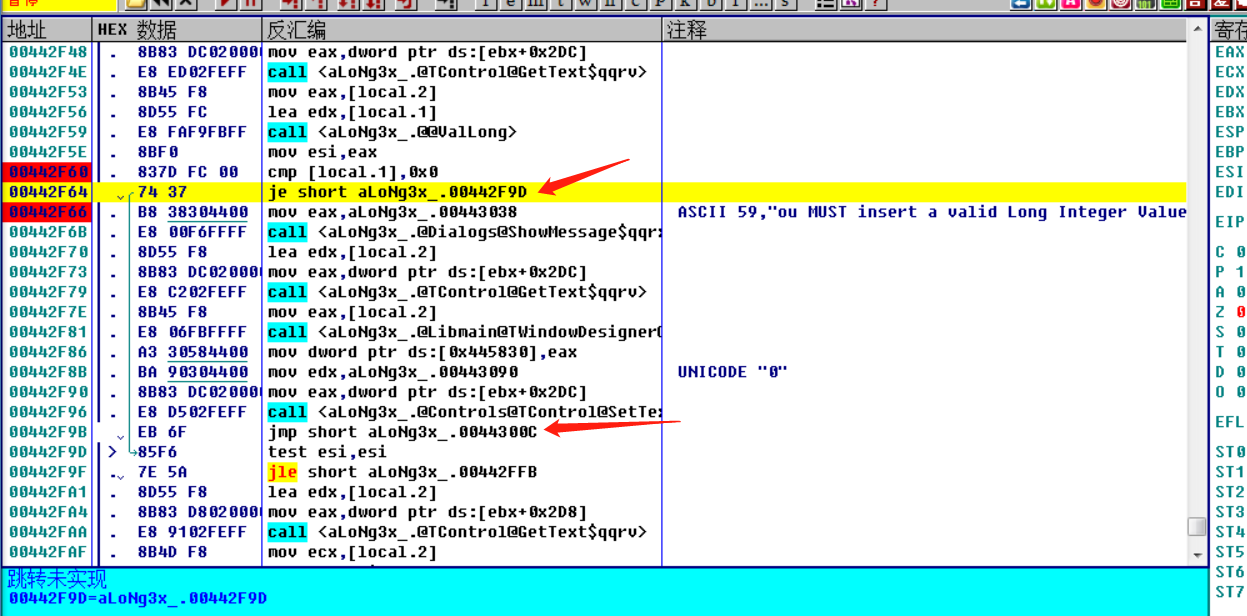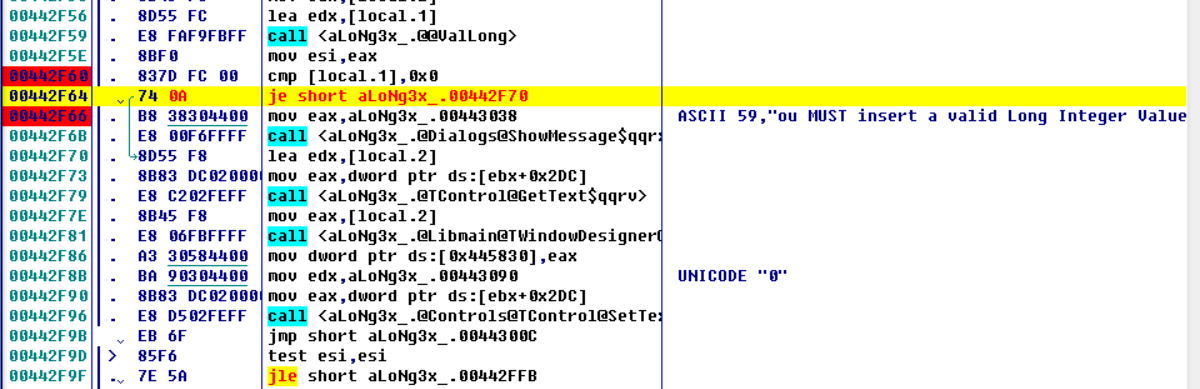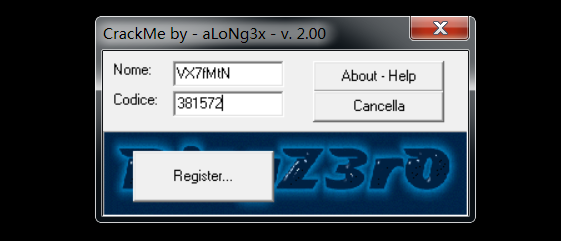ps:其实双击指令位置改就行hhhh，没必要自己计算硬编码

### End